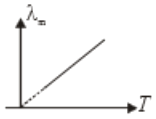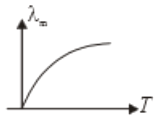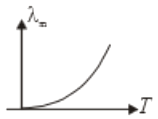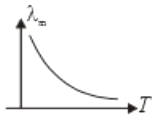One kilogram of ice at $0°$C is mixed with one kilogram of water at 80$°C$. The final temperature of the mixture is (Take: Specific heat of water = 4200 J $k{g}^{-1}{k}^{-1}$, Latent heat of ice = 336 kJ $k{g}^{-1}$)

(1) $0°$C              (2) $50°C$

(3) $40°C$           (4) $60°C$

Concept Questions :-

Calorimetry
NEET 2021 - Achiever Batch - Aryan Raj Singh

Difficulty Level:

The fastest mode of heat transfer is

1.  Conduction

3.  Convection

4.  all are  equally fast

Concept Questions :-

Temperature scales
NEET 2021 - Achiever Batch - Aryan Raj Singh

Difficulty Level:

1.  310.9

2.  37.8

3.  100

4.  122.4

Concept Questions :-

Temperature scales
NEET 2021 - Achiever Batch - Aryan Raj Singh

Difficulty Level:

A black body at temperature 300K radiates heat at the rate E. If its temperature is increased by 600K, the rate of radiation will increase to -

1.  16E

2.  64E

3.  81E

4.  256E

Concept Questions :-

NEET 2021 - Achiever Batch - Aryan Raj Singh

Difficulty Level:

1.  Less than 5 minutes

2.  equal to 5 minutes

3.  More than 5 minutes

4.  Can't say anything like the temperature of surrounding is not known

Concept Questions :-

Newton's law of cooling
NEET 2021 - Achiever Batch - Aryan Raj Singh

Difficulty Level:

If ${\lambda }_{m}$ is the wavelength, corresponding to which the radiant intensity of a block is maximum and its absolute temperature is T. Which of the following graph correctly represents the variation of ${\lambda }_{m}$ with T.

1.2.3.4.Concept Questions :-

Wien displacement law
NEET 2021 - Achiever Batch - Aryan Raj Singh

Difficulty Level:

Heat capacity is equal to the product of:

1.  mass and gas constant

2.  mass and specific heat

3.  latent heat and volume of water

Concept Questions :-

Calorimetry
NEET 2021 - Achiever Batch - Aryan Raj Singh

Difficulty Level:

When a block of iron floats in Hg at $0°C$, a fraction ${K}_{1}$ of its volumen= is submerged, while at temperature of $60°C$ a fraction ${K}_{2}$ is seen to be submersed. If the coefficient of volume expansion of iron is ${\gamma }_{Fe}$ and that of mercury is ${\gamma }_{Hg}$, then the ratio $\frac{{K}_{1}}{{K}_{2}}$ can be expressed as:

(1) $\frac{1+60{\gamma }_{Fe}}{1+60{\gamma }_{Hg}}$

(2) $\frac{1-60{\gamma }_{Fe}}{1+60{\gamma }_{Hg}}$

(3) $\frac{1+60{\gamma }_{Fe}}{1-60{\gamma }_{Hg}}$

(4) $\frac{1+60{\gamma }_{He}}{1-60{\gamma }_{Fe}}$

Concept Questions :-

Thermal expansion
NEET 2021 - Achiever Batch - Aryan Raj Singh

Difficulty Level:

Hot water cools from 60$°\mathrm{C}$ to 50$°\mathrm{C}$ in first 10 minutes and from 50$°\mathrm{C}$ to 42$°\mathrm{C}$ in next 10 minutes. The temperature of surrounding is :

1.

2.

3.

4.  $20°\mathrm{C}$

Concept Questions :-

Newton's law of cooling
NEET 2021 - Achiever Batch - Aryan Raj Singh

Difficulty Level:

1.

2.

3.

4.  $33°\mathrm{C}$

Concept Questions :-

Thermal expansion